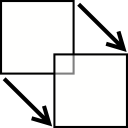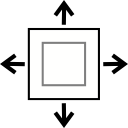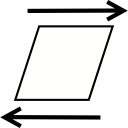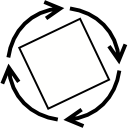# Matrix Math Tutorial

2014-01-23

The subject of this tutorial is 4x4 transformation matrices. While not essential for basic 3D work, this information can be very useful for more advanced purposes. Familiarity with Vector Math and Quaternion Math is recommended before reading.

## Definition

A transformation matrix is a map from one coordinate system to another. It is a "recipe" for a transformation, such as rotation, translation, scaling, and many other things. You can multiply a vector by a matrix in order to perform the matrix's transformation on it, remapping it from its original coordinate system into the new one represented by the matrix. Matrices can be used to change the size, shape, orientation, and/or position of geometry in your 3D world.

A matrix is typically represented as an array of 16 floating-point numbers. Whether to use a one-dimensional or a two-dimensional array is a matter of personal preference. This tutorial uses a flat array of 16 floats in the following struct to represent a matrix:

```typedef struct Matrix { float m; } Matrix; ```

Regardless of the type of array you choose, you can think of a matrix as a 4x4 grid of floating point numbers. The first three columns can be visualized as direction vectors on each axis. The fourth column contains a spatial offset on each axis. There is a matrix known as the identity matrix which has particular significance, as it performs no transformation at all; multiplying anything by it gives the same result unchanged. The identity matrix looks like this:

 1 0 0 0 0 1 0 0 0 0 1 0 0 0 0 1

This function loads the identity matrix.

```void Matrix_loadIdentity(Matrix * matrix) { matrix->m = 1.0f; matrix->m = 0.0f; matrix->m = 0.0f; matrix->m = 0.0f; matrix->m = 0.0f; matrix->m = 1.0f; matrix->m = 0.0f; matrix->m = 0.0f; matrix->m = 0.0f; matrix->m = 0.0f; matrix->m = 1.0f; matrix->m = 0.0f; matrix->m = 0.0f; matrix->m = 0.0f; matrix->m = 0.0f; matrix->m = 1.0f; } ```

We'll look at the identity matrix in greater detail in a moment, but first a note about matrix layout. There are two ways to index the values in a matrix: row-major and column-major. Row-major matrices are indexed first left-to-right, then top-to-bottom; column-major matrices are top-to-bottom first, left-to-right second.

A row-major matrix (used by Direct3D) is indexed like this:

 0 1 2 3 4 5 6 7 8 9 10 11 12 13 14 15

A column-major matrix (used by OpenGL, and throughout this tutorial) is indexed like this:

 0 4 8 12 1 5 9 13 2 6 10 14 3 7 11 15

You can convert between these two matrix representations by transposing the matrix. A transpose mirrors the matrix values diagonally. Here's function that transposes a matrix (using a convenience macro for initializing a Matrix struct):

```#define MATRIX(m0, m4, m8, m12, \ m1, m5, m9, m13, \ m2, m6, m10, m14, \ m3, m7, m11, m15) \ ((Matrix) {{m0, m1, m2, m3, \ m4, m5, m6, m7, \ m8, m9, m10, m11, \ m12, m13, m14, m15}}) void Matrix_transpose(Matrix * matrix) { *matrix = MATRIX(matrix->m, matrix->m, matrix->m, matrix->m, matrix->m, matrix->m, matrix->m, matrix->m, matrix->m, matrix->m, matrix->m, matrix->m, matrix->m, matrix->m, matrix->m, matrix->m); } ```

## Usage

Matrices are most commonly used for five specific transformations - translation, rotation, scaling, shearing, and perspective. Translation is simply a spatial offset; movement from one location to another. Rotation takes place on an arbitrary axis, and is applied in a single operation; gimbal lock is not a problem with matrices. Scaling can take place on any axis independently, or uniformly on all axes. Shearing can be thought of as applying a slant; for example, in italicized text, the Y axis is sheared by a positive X value. Perspective is used to create the illusion of depth, making distant objects appear smaller. A single matrix can perform any and all of these operations simultaneously.

For each transformation, there is a set of cells in the matrix that are significant to it. When you want to apply one of these operations to a matrix, you'll generally start with the identity matrix, insert the appropriate values into the cells that are significant to the operation, and multiply the current matrix by it.

## Multiplication

Recall that a transformation matrix is a map from one coordinate system to another. By multiplying a vector by a matrix, you apply the matrix's transformation to the vector, thereby mapping the vector from its original coordinate system to the one expressed by the matrix.

Matrices can be multiplied with each other, which has the effect of concatenating their transformations together. Example: `matrix1` is a rotation matrix that rotates by 90° on the Z axis. `matrix2` is a scaling matrix that scales by a factor of 2. Multiplying `matrix1` by `matrix2` (or `matrix2` by `matrix1`; see below) results in `matrix3`, which simultaneously scales by a factor of 2 and rotates by 90° on the Z axis.

Matrix multiplication is not commutative - that is, the order of the multiplication may make a difference in the result. Example: `matrix1` rotates 180° on the Y axis. `matrix2` translates 4 units on the X axis. `matrix1 * matrix2` results in `matrix3`. `matrix2 * matrix1` results in `matrix4`, which performs a different transformation from `matrix3`. In `matrix3`, the rotation affects the subsequent translation, and it will translate in the opposite direction as `matrix4`.

When multiplying two matrices together, the value of each cell in the result matrix is computed as follows: Each value on the cell's row in the left-hand matrix (starting from the left) is multiplied by each value in the cell's column in the right-hand matrix (starting from the top). The results of the multiplications are added together, and the sum becomes the new value at that cell in the matrix.

 2 6 10 14
matrix1
 4 5 6 7
matrix2
 sum
result

Here's an example function that multiplies two matrices together:

```void Matrix_multiply(Matrix * matrix1, Matrix m2) { Matrix m1, result; int cellIndex, cellRow, cellColumn, rowAndColumnIndex; m1 = *matrix1; for (cellIndex = 0; cellIndex < 16; cellIndex++) { result.m[cellIndex] = 0.0f; cellRow = cellIndex % 4; cellColumn = cellIndex / 4; for (rowAndColumnIndex = 0; rowAndColumnIndex < 4; rowAndColumnIndex++) { result.m[cellIndex] += m1.m[cellRow + (rowAndColumnIndex * 4)] * m2.m[(cellColumn * 4) + rowAndColumnIndex]; } } *matrix1 = result; } ```

Here's a function that does the same thing without any loops. This function is used in the reference implementation at the end of this tutorial; the above function is included only for readability.

```void Matrix_multiply(Matrix * matrix1, Matrix m2) { Matrix m1, result; m1 = *matrix1; result.m = m1.m * m2.m + m1.m * m2.m + m1.m * m2.m + m1.m * m2.m; result.m = m1.m * m2.m + m1.m * m2.m + m1.m * m2.m + m1.m * m2.m; result.m = m1.m * m2.m + m1.m * m2.m + m1.m * m2.m + m1.m * m2.m; result.m = m1.m * m2.m + m1.m * m2.m + m1.m * m2.m + m1.m * m2.m; result.m = m1.m * m2.m + m1.m * m2.m + m1.m * m2.m + m1.m * m2.m; result.m = m1.m * m2.m + m1.m * m2.m + m1.m * m2.m + m1.m * m2.m; result.m = m1.m * m2.m + m1.m * m2.m + m1.m * m2.m + m1.m * m2.m; result.m = m1.m * m2.m + m1.m * m2.m + m1.m * m2.m + m1.m * m2.m; result.m = m1.m * m2.m + m1.m * m2.m + m1.m * m2.m + m1.m * m2.m; result.m = m1.m * m2.m + m1.m * m2.m + m1.m * m2.m + m1.m * m2.m; result.m = m1.m * m2.m + m1.m * m2.m + m1.m * m2.m + m1.m * m2.m; result.m = m1.m * m2.m + m1.m * m2.m + m1.m * m2.m + m1.m * m2.m; result.m = m1.m * m2.m + m1.m * m2.m + m1.m * m2.m + m1.m * m2.m; result.m = m1.m * m2.m + m1.m * m2.m + m1.m * m2.m + m1.m * m2.m; result.m = m1.m * m2.m + m1.m * m2.m + m1.m * m2.m + m1.m * m2.m; result.m = m1.m * m2.m + m1.m * m2.m + m1.m * m2.m + m1.m * m2.m; *matrix1 = result; } ```

## Multiplying vectors

Each of these functions is used to multiply a vector by a matrix. Since the implementation is slightly different for each case, I'm including functions for multiplying 2-component (XY), 3-component (XYZ), and 4-component (XYZW) vectors. The reference implementation at the bottom of this tutorial currently only implements 3-component vector multiplication.

```Vector2f Matrix_multiplyVector2f(Matrix matrix, Vector2f vector) { Vector2f result; result.x = matrix.m * vector.x + matrix.m * vector.y + matrix.m; result.y = matrix.m * vector.x + matrix.m * vector.y + matrix.m; return result; } Vector3f Matrix_multiplyVector3f(Matrix matrix, Vector3f vector) { Vector3f result; result.x = matrix.m * vector.x + matrix.m * vector.y + matrix.m * vector.z + matrix.m; result.y = matrix.m * vector.x + matrix.m * vector.y + matrix.m * vector.z + matrix.m; result.z = matrix.m * vector.x + matrix.m * vector.y + matrix.m * vector.z + matrix.m; return result; } Vector4f Matrix_multiplyVector4f(Matrix matrix, Vector4f vector) { Vector4f result; result.x = matrix.m * vector.x + matrix.m * vector.y + matrix.m * vector.z + matrix.m * vector.w; result.y = matrix.m * vector.x + matrix.m * vector.y + matrix.m * vector.z + matrix.m * vector.w; result.z = matrix.m * vector.x + matrix.m * vector.y + matrix.m * vector.z + matrix.m * vector.w; result.w = matrix.m * vector.x + matrix.m * vector.y + matrix.m * vector.z + matrix.m * vector.w; return result; } ```

## Translation

A translation matrix is very simple. The spatial offset on each axis is assigned to a cell in the fourth column. When a vector is multiplied by it, this has an effect equivalent to adding each translation value to its corresponding vector component.Translation
 1 0 0 X axisoffset 0 1 0 Y axisoffset 0 0 1 Z axisoffset 0 0 0 1.0

This function translates a matrix.

```void Matrix_translate(Matrix * matrix, float x, float y, float z) { Matrix translationMatrix; Matrix_loadIdentity(&translationMatrix); translationMatrix.m = x; translationMatrix.m = y; translationMatrix.m = z; Matrix_multiply(matrix, translationMatrix); } ```

## Scaling

A scaling matrix has a scale multiplier for each axis. Essentially, it adjusts the length of the direction vectors in the first three columns:Scaling
 X axisscale 0.0 0.0 0 0.0 Y axisscale 0.0 0 0.0 0.0 Z axisscale 0 0.0 0.0 0.0 1

This function can be used to scale a matrix.

```void Matrix_scale(Matrix * matrix, float x, float y, float z) { Matrix scalingMatrix; Matrix_loadIdentity(&scalingMatrix); scalingMatrix.m = x; scalingMatrix.m = y; scalingMatrix.m = z; Matrix_multiply(matrix, scalingMatrix); } ```

## Shearing

For each axis in a shearing matrix, there are two values that correspond to the other two axes. For each unit on that axis, coordinates will move as many units as specified on the other two axes. For example, if the Y axis is sheared by 1.0 on the X axis, coordinates on the Y axis will slant at a 45° angle in the direction of the X axis.Shearing
 1.0 Y axis X shear Z axis X shear 0 X axis Y shear 1.0 Z axis Y shear 0 X axis Z shear Y axis Z shear 1.0 0 0.0 0.0 0.0 1

This set of functions can be used to shear a matrix. The axis in the function name is sheared by the axes in its parameter list.

```void Matrix_shearX(Matrix * matrix, float y, float z) { Matrix shearingMatrix; Matrix_loadIdentity(&shearingMatrix); shearingMatrix.m = y; shearingMatrix.m = z; Matrix_multiply(matrix, shearingMatrix); } void Matrix_shearY(Matrix * matrix, float x, float z) { Matrix shearingMatrix; Matrix_loadIdentity(&shearingMatrix); shearingMatrix.m = x; shearingMatrix.m = z; Matrix_multiply(matrix, shearingMatrix); } void Matrix_shearZ(Matrix * matrix, float x, float y) { Matrix shearingMatrix; Matrix_loadIdentity(&shearingMatrix); shearingMatrix.m = x; shearingMatrix.m = y; Matrix_multiply(matrix, shearingMatrix); } ```

## Rotation

In a rotation matrix, the direction vectors in the first three columns are uniformly rotated to a new orientation. The difference between this orientation and the identity matrix is the same as the rotation the matrix performs. The three vectors are of unit length and are orthogonal to each other.Rotation
 X axis X coord Y axis X coord Z axis X coord 0 X axis Y coord Y axis Y coord Z axis Y coord 0 X axis Z coord Y axis Z coord Z axis Z coord 0 0.0 0.0 0.0 1

This function rotates a matrix. A quaternion is used for the rotation; more information on quaternions is available in the Quaternion tutorial. The `angle` is represented as radians.

```void Matrix_rotate(Matrix * matrix, Vector3f axis, float angle) { Matrix rotationMatrix; Quaternion quaternion; quaternion = Quaternion_fromAxisAngle(axis, angle); rotationMatrix = Quaternion_toMatrix(quaternion); Matrix_multiply(matrix, rotationMatrix); } ```

## Perspective

A perspective projection matrix uses the W coordinate of the Z axis to make objects in the distance appear smaller to create the illusion of depth.Perspective
 X axisscale 0.0 0.0 0.0 0.0 Y axisscale 0.0 0.0 0.0 0.0 Clipdistance Clipdistance 0.0 0.0 Perspectiveforeshortening 0.0

This function sets up a perspective projection matrix. The `fovY` parameter is the angle of the field of view on the Y axis, in degrees. The `aspect` is the ratio of width to height of the projection. `zNear` and `zFar` specify the near and far clipping planes, respectively. This function works the same way as OpenGL's `gluPerspective` function.

```void Matrix_applyPerspective(Matrix * matrix, float fovYDegrees, float aspect, float zNear, float zFar) { Matrix perspectiveMatrix; float sine, cotangent, deltaZ; fovYDegrees = fovYDegrees * M_PI / 360.0f; deltaZ = zFar - zNear; sine = sin(fovYDegrees); if (deltaZ == 0.0f || sine == 0.0f || aspect == 0.0f) { return; } cotangent = cos(fovYDegrees) / sine; Matrix_loadIdentity(&perspectiveMatrix); perspectiveMatrix.m = cotangent / aspect; perspectiveMatrix.m = cotangent; perspectiveMatrix.m = -(zFar + zNear) / deltaZ; perspectiveMatrix.m = -1.0f; perspectiveMatrix.m = -2.0f * zNear * zFar / deltaZ; perspectiveMatrix.m = 0.0f; Matrix_multiply(matrix, perspectiveMatrix); } ```

## Inversion

In most cases, a transformation matrix can be inverted. A matrix performs the exact opposite transformation when inverted, such that `vector * matrix * inverse(matrix) == vector`, and `matrix * inverse(matrix) == Matrix_identity()`.

To compute the inverse of a matrix, you first have to calculate the matrix's determinant value. The determinant of a matrix is a floating point value which is used to indicate whether the matrix has an inverse or not, and is used in the inverse computation itself. As long as the determinant is nonzero, an inverse exists and can be computed. I won't go into detail about the math used to compute the determinant, but see Q24 (and Q23) in the Matrix & Quaternion FAQ if you're curious. Meanwhile, here's an implementation:

```static float Matrix_subdeterminant(Matrix matrix, int excludeIndex) { int index4x4, index3x3; float matrix3x3; if (excludeIndex < 0 || excludeIndex > 15) { return 1.0f; } index3x3 = 0; for (index4x4 = 0; index4x4 < 16; index4x4++) { if (index4x4 / 4 == excludeIndex / 4 || index4x4 % 4 == excludeIndex % 4) { continue; } matrix3x3[index3x3++] = matrix.m[index4x4]; } return matrix3x3 * (matrix3x3 * matrix3x3 - matrix3x3 * matrix3x3) - matrix3x3 * (matrix3x3 * matrix3x3 - matrix3x3 * matrix3x3) + matrix3x3 * (matrix3x3 * matrix3x3 - matrix3x3 * matrix3x3); } float Matrix_determinant(Matrix matrix) { float subdeterminant0, subdeterminant1, subdeterminant2, subdeterminant3; subdeterminant0 = Matrix_subdeterminant(matrix, 0); subdeterminant1 = Matrix_subdeterminant(matrix, 4); subdeterminant2 = Matrix_subdeterminant(matrix, 8); subdeterminant3 = Matrix_subdeterminant(matrix, 12); return matrix.m * subdeterminant0 + matrix.m * -subdeterminant1 + matrix.m * subdeterminant2 + matrix.m * -subdeterminant3; } ```

With the determinant computed, we can now compute the inverse of the matrix, assuming the determinant is non-zero. Note that this function performs no checking of the determinant; using it with a matrix that has a determinant of zero will give you a matrix full of `nan` and/or `inf` values.

```void Matrix_invert(Matrix * matrix) { float determinant; Matrix result; int index, indexTransposed; int sign; determinant = Matrix_determinant(*matrix); for (index = 0; index < 16; index++) { sign = 1 - (index % 4 + index / 4) % 2 * 2; indexTransposed = index % 4 * 4 + index / 4; result.m[indexTransposed] = Matrix_subdeterminant(*matrix, index) * sign / determinant; } *matrix = result; } ```

## More on the identity matrix

If you look at the identity matrix again, you'll notice that, neatly enough, the first three columns are unit vectors that point right, up, and forward, respectively. The fourth column is the translation vector; it contains all zeros in the first three rows, because the identity matrix performs no translation.

The values in the bottom row are more abstract, and can't be explained as simply as the others. I recommend using the Matrix playground application (linked at the bottom of this tutorial) to experiment with these values in order to understand how they affect transformations.

 1 0 0 0 0 1 0 0 0 0 1 0 0 0 0 1

If you'd like to know more, I recommend that you read the Matrix & Quaternion FAQ. It was a great help to me while writing this tutorial, and is an excellent resource for everything relating to matrices and quaternions. Feel free to contact me with any questions you might have, and I'll get back to you as quickly as possible.